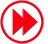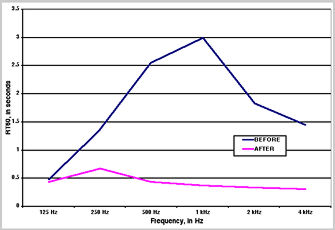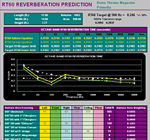# CUSTOM INSTALLATION HOW-TO

## Design the Ultimate Home Theater– On a Budget Page 7

Tip #4: Calculating RT60 (Without Test Equipment)

RT60 defines the amount of time it takes an impulse sound at a given frequency to decay by 60 dB and is typically used in large rooms. In small rooms, you can use RT60 calculations to get a general idea of the room's reverberant character. The point is to lower this reverberation level evenly across the entire frequency range without making the room overly dead. To calculate RT60, you must select an ideal reverberation time to aim for, and you need to know the absorption characteristics of various materials at various frequencies, the surface area of those materials, and your total room volume.

Calculating your ideal reverberation time requires a little experience with a scientific calculator. The formula is as follows: 0.3 times the cube root of (volume of your room/3,532)Determine your room's volume by multiplying the height by the width by the length, then divide that number by 3,532. If your calculator doesn't have a cube-root function, you can use the "y to the x power" function. With this, use the previous total for y and use 0.333 for x. The calculations for our room came to about 0.25 seconds.

Next, you need to find the absorption characteristics of the materials in your room. This may be easier said than done, as they're not usually listed with the products at your local hardware store, and the government document called the Compendium of Materials for Noise Control (NIOSH #80-116) is now out of print. We've listed the absorption characteristics for basic materials in figure 1. You may also find them in acoustic texts like F. Alton Everest's The Master Handbook of Acoustics. The formula is simple: Multiply the material's absorption characteristic at each frequency by the material's surface area, in square feet, to get the subtotal of absorption for that material (in sabins). Then add all of the subtotals for the room's different materials at each given frequency. Multiply the room's total volume by a constant (0.049) and divide that number by the total absorption figure.The problem with these calculations is that they are only as accurate as the number of surfaces accounted for. For example, it's not likely that the entire carpet is exposed, so you have to subtract a certain amount for those areas of the carpet that are covered with other items. Then you have to calculate the absorption of those items, assuming that you can find absorption specifications for them. Also, some materials may partly obscure others. Since 1-inch-thick Fiberglas mostly absorbs frequencies above about 750 Hz, frequencies below this point travel through to the material behind the Fiberglas. Therefore, you'll need to figure that the drywall that's covered by the Fiberglas will retain its absorption characteristics up to about 750 Hz. In most cases, the most difficult frequencies to absorb will be below 500 Hz. We may discuss options for these frequencies in a future issue.As part of their home theater design, A/V Room Service, Russ Herschelmann Designs, and Performance Media Industries calculate more-precise reverberation times and create acoustic-material layout plans. We followed PMI's suggestions for our room (see figure 2 for the layout plan and figure 3 for reverberation times). If you do the calculations on your own, remember that the Sabine equation creates crude estimates that you should only use as a guide. They can at least give you an idea of how various materials react to sound. You can always experiment from there.ARTICLE CONTENTSX